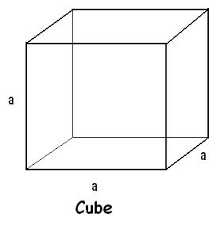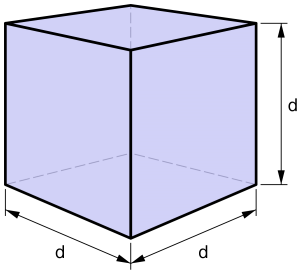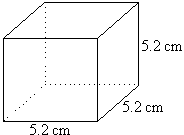## How to Calculate and Solve for the Length of a Side and Perimeter of a Cube | The Calculator EncyclopediaThe image above is a cube.

To compute the perimeter of a cube, one essential parameter is needed and this parameter is length of one of the sides (a).

The formula for calculating the perimeter of a cube:

P = 12a

Where;
P = Perimeter of the cube
a = Length of one of the sides of the cube

Let’s solve an example;
Find the perimeter of a cube when the length of one of the sides is 14 cm.

This implies that;
a = Length of one of the sides = 14 cm

P = 12a
P = 12 x 14
P = 168

Therefore, the perimeter of the cube is 168 cm.

Calculating the Length of one of the sides of a Cube using the Perimeter of the Cube.

a = P / 12

Where;
a = Length of one of the sides of the cube
P = Perimeter of the cube

Let’s solve an example;
Find the length of one of the sides of a cube when the perimeter of the cube is 200 cm.

This implies that;
P = Perimeter of the cube = 200 cm

a = P / 12
a = 200 / 12
a = 16.67

Therefore, the length of one of the sides is 16.67 cm.

## How to Calculate and Solve for the Volume and Length of a Cube | The Calculator EncyclopediaThe image above is a cube.

To compute the volume of a cube, one essential parameter is needed and this parameter is the length of the cube (l).

The formula for calculating the volume of a cube is;

V = l3

Where;

V = Volume of a cube
l = Length of a cube

Let’s solve an example:
Find the volume of a cube where the length of a cube is 4 cm.

This implies that;
l = length of the cube = 4 cm.

V = l3
V = 43
V = 64 cm3

Therefore, the volume of the cube is 64 cm3

Calculating the Length of a cube using the Volume of the cube.

The formula is l = 3√V

Where;

V = Volume of a cube
l = length of a cube

Let’s solve an example:
Find the length of a cube where the volume of the cube is 120 cm3

This implies that;
V = Volume of the cube = 120 cm3

l = 3√V
l = 3√120
l = 4.93

Therefore, the length of the cube is 4.93 cm.

## How to Calculate and Solve for the Centre of Gravity of a CubeThe image above is a cube with a length of 5.2 cm.
To compute the centre of gravity of a cube, one essential parameter is needed and this parameter is the length of the cube (l).

The formula for calculating the centre of gravity of a cube is:

C.G. = 0.5(l)

Where:
l = Length of the Cube
C.G. = Centre of Gravity

Let’s solve an example:
Find the centre of gravity of a cube where the length of a side of the cube is 5.2 cm.

This implies that:
l = Length of the Cube = 5.2